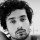Software Testing Squad
All About Software Testing In One Place!
131 Members
Join this group to post and comment.Gaurav Prasad
Computer Science
15 Jan 2019

# What is cyclomatic complexity?

explain its uses also?Rohit Kumar
Electronic
4mos ago

It is software metric to measure the complexity of the program for coding related errors. In software terms ,  It is a quantitative measure of the number of linearly independent paths through a program's source code.

The software was  developed by Thomas J. McCabe Sr. in 1976.

It is important and used for two different reasons :

1. To limit code complexity
2. It also determines number of test cases required

These metric is said to be the most complicated metric to understand, thus making it difficult to calculate.

`//------------------ `

To calculate CC, following formula is use:

CYC = E – N + 2P

In this equation:

P = number of disconnected parts of the flow graph (e.g. a calling program and a subroutine)

E = number of edges (transfers of control)

N = number of nodes (sequential group of statements containing only one transfer of control)

`//------------------ `Gaurav Prasad
Computer Science
4mos ago

Thanks for your contribution.